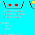# SIMPLE ALGEBRAIC OPERATION

We already knew about arithmetical operation of addition, subtraction, multiplication, division and exponentiation on integer and frcation.

Example 1:

1. Find the result of the following addition and subtraction!
a. -19 + 7
b. 18 - 5
c. -23 - 7

2. Find the result of the following multiplication and division!
a. 7 x (-19)
b. -11 x 20
c. 14 x -89

These subject as foundation for understanding  about algebraic expression operation, include simplify an algebraic expression.

Example 2:
Simplify the following expression!
a. 4x + 6x
b. 7y - 2y
c. 8c + 9b - 5b - 4c
d. 5p - 4q - 3q + 7p

a. 10x
b. 5y
c. 4b+4c
d. 12p -7q

### 2 Komentar di Postingan "SIMPLE ALGEBRAIC OPERATION "

1.just blogwalking in a moment to read this wonderful article :)

1.thanks for visit

Untuk menyisipkan kode,gunakan tag
[code]KODE ANDA[/code]
Untuk menyisipkan quote,gunakan tag
[blockquote]KATA-KATA ANDA[/blockquote]
Untuk menyisipkan gambar,gunakan tag
[img]URL GAMBAR ANDA[/img]
Untuk menyisipkan video,gunakan tag